/

### A potential way in which the Central Limit Theorem (CLT) can break down via combinations of lots of independent gamma distributed random variables

The Gamma distribution has the the ‘summation’ property that if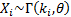for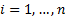and the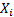are independent then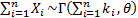.

So, suppose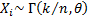. Then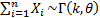. Thus we appear to be combining more and more (independent) random variables each of which has smaller and smaller mean,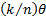, and variance,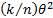, so we might expect the Central Limit Theorem (CLT) to apply.

In fact, for the CLT to apply we need somewhat more onerous regularity conditions to be satisfied, including a focus on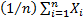and (usually) that the distributions of thedo not change aschanges (as well as being of finite variance). The above example does not satisfy these amplified regularity conditions because aschanges the distribution of eachchanges. Although the means (and variances) get smaller and smaller (which you would have thought would help with satisfying the CLT), each individualbecomes more and more skewed and has a greater and greater (excess) kurtosis, see e.g. the Nematrian webpage on the Gamma distribution.